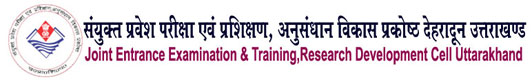Welcome to IRDT Uttarakhand E-Syllabus Web Portal05 COMPUTER SCIENCE & ENGGINEERING
 Duration : 03 Year Eligibility : 10th with Science & Mathematics Intake : 25/40/60(As approved by AICTE/State Govt.) Pattern of the Examination : Semester System Assessment Type : The assessment will we carry out through credit point system/Learning Based Out comes Theory & Practical Ratio : 50: 50 Industrial Training : Included after IInd Semester Ecology & Environment : As pe Government Directions a subject named Environmental Science & Energy Management has been included. Entrepreneurship Development : A subject on Entrepreneurship Development has been incorporated after IInd Semester. General Proficiency : A provision of 6 periods per week has been made. It Included of various cocurricular activities like games, hobby clubs, seminars, declamation contests, extension lectures, NCC, NSS, cultural activities and discipline etc.

### Syllabus

Paper Code :
991002
Paper Name :
APPLIED MATHEMATICS-I

#### Unit – I (Algebra) [ 25 Periods ]

1.1- Value of nP & nC (Without proof), Binomial theorem-(without proof) for r r
positive integral index (expansion, general term, middle term) and for any index
(expansion).
1.2-Partial fractions- (linear factors, repeated linear factors, non reducible quadratic
factors only).
1.3-Determinants-Definition, Properties of determinants, Expansion of determinants
(of order 2 and 3), Solution of simultaneous equations using Cramer’s rule ( in 2
and 3 unknowns ).
1.4-Matrices- Definition of matrix, addition, subtraction, multiplication of matrices (
upto 3 order), singular and non singular matrices, Adjoint of a matrix, Inverse of
a matrix by adjoint method (up to 3x 3 only).
1.5-Sets: Sets and their representation, Empty set, finite and infinite sets, equal sets,
subsets, power set, universal set, operation on sets, complement of set.

#### Unit – II (Trigonometry [15 Periods]

1.1-Review of ratios of some standard angles (0,30,45,60,90 degrees), T-Ratios of
Allied angles (without proof), Sum, difference formulae and their applications
(without proof). Product formulae (Transformation of product to sum, difference
and vice versa). T-Ratios of multiple angles, sub-multiple angles (2A, 3A, A/2).

#### Unit – III (Complex Number) [ 10 Periods ]

1.1 Definition of complex numbers,Real and imaginary parts,Polar and Cartesian form and their conversion,Conjugate,Modulus and argument of a complex Number,Addition,Subtraction,Multiplication,division of complex numbers.
1.2 De-Moiver's Theorem(Statement Only) related simple problems,rth root of unity.

#### Unit – IV (Differential Calculus) [ 30 Periods ]

1.1- Function: Definitions of variables, constants, open & closed intervals.
1.2- Definitions & types of functions-simple examples.
1.3- Concept & definition of Limit.
1.4- Standard limits of algebraic, trigonometric, exponential & logarithmic
functions- simple problems. Examine the Continuity of a function at any point
(simple problem only).
1.5 Examine the continuity of a function at any point(Simple Problam Only)
1.6-Differentiation by (first principal) or by definition of differention xn, sin x, cos
x, tan x, ex, log x only . a
1.7-Differentiation of sum, product and quotient of functions. Differentiation of
function of a function.
1.8-Differentiation of trigonometric inverse functions. Logarithmic differentiation.
Exponential differentiation, Successive differentiation (up to third term only).
1.9-Application (a) Maxima and minima (b) Equation of tangent and normal to a
curve (for explicit functions only) (c) L’ Hospital rule for solving in in
determinate form ( 0/0, )

#### REFERENCE BOOK/ TEXT BOOK

1-HIGHER ALGEBRA BY HALL & KNIGHT

2-PLANE TRIGNOMETRY BY S L LONEY

3-ENGINEERING MATHEMATICS BY  SASTRY (PHI Learning)

4-ENGINEERING MATHEMATICS BY B S GREWAL  (Khanna Publishers)

5-ENGINEERING MATHEMATICS BY A.B MATHUR  (Khanna Publishers)

6-APPLIED MATHMATICS , BY  M K KANYAL   (Khanna Publishers, New Delhi)

7-APPLIED MATHEMATICS-I ,BY DR A.K.SINHA,Satya Prakashan,New Delhi

8-ENGINEERING MATHEMATICS,BY C.B.GUPTA,S.R.SINGH,MUKESH KUMAR,Mc GRAW HILL EDUCATION.

9-APPLIED MATHEMATICS BY R.D.SHARMA,DHANPAT RAI PUBLICATIONS,NEW DELHI

10-ENGINEERING MATHEMATICS,VOL-I & II,BY S.S.SABRWAL AND SUNITA JAIN,EAGLE PRAKASHAN,JALANDHER

11-BASIC ENGINEERING MATHEMATICS,BY JHON BIRD,NEWNES PUBLICATIONS.

12-A TEXT BOOK OF ENGINEERING MATHEMATICS,BY A.GANESH,G.BALASUBRAMNIUM.

13-POLYTECHNIC MATHEMATICS,BY DR. D.S.PRAKASH,S.CHAND,PUBLICATIONS,NEW DELHI.

14-A TEXT BOOK OF ENGINEERING MATHEMATICS,BY N.P.BALI & DR. MANISH GOYAL,KINDLY PUBLICATION.

15-ENGINEERING MATHEMATICS,BY C.B.GUTA,S.R.SINGH & MUKESH KUMAR,Mc GRAW-HILL PUBLICATIONS,DELHI

16-APPLIED MATHEMATICS, BY KAPOOR,NAV DISTRIBUTOR,MEERUT.

Website Developed By Softmaart